##### Statistics: 1001 Practice Problems For Dummies (+ Free Online Practice)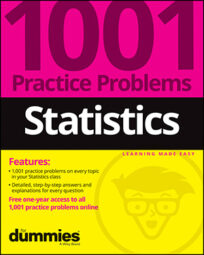In the following practice problems, you will be connecting a population proportion to a survey. A website ran a random survey of 200 customers who purchased products online in the past 12 months. The survey found that 150 customers were "very satisfied."

## Sample questions

1. What are the sample proportion and the standard error for the sample proportion, based on this data?

Find the sample proportion,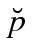by dividing the number of "successes" (75 in this case) by the sample size (200):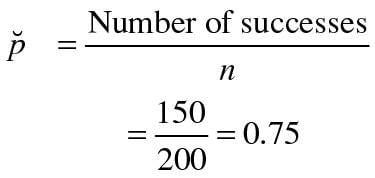The sample proportion represents the proportion of customers in the sample who are satisfied with their online purchases.

Then use the following formula to find the standard error (SE):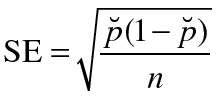whereis the sample proportion and n is the sample size: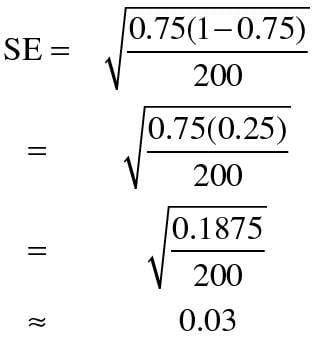So the standard error for the sample proportion in this example is 0.03.

2. With a 95% confidence level, what is the margin of error for the estimate of the proportion of all customers who purchased products online in the past 12 months?

Use the formula for finding the margin of error (MOE):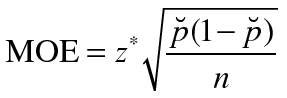is the sample proportion, n is the sample size, and z* is the appropriate value from the standard normal distribution for your desired confidence level. For a 95% confidence level, the z*-value is 1.96. Note that the margin of error is the z*-value times the standard error.

Now, plug in the known values and solve: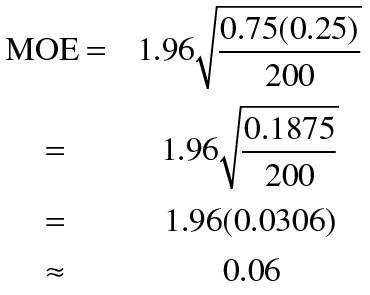With 95% confidence, the margin of error is plus/minus 0.06 for estimating the proportion of all customers who purchased products online in the past 12 months.

If you need more practice on this and other topics from your statistics course, visit 1,001 Statistics Practice Problems For Dummies to purchase online access to 1,001 statistics practice problems! We can help you track your performance, see where you need to study, and create customized problem sets to master your stats skills.